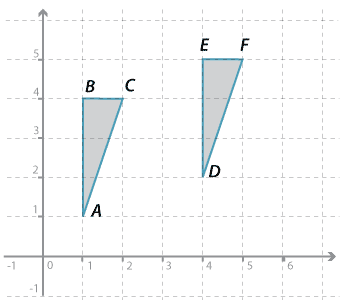#### Question 1Detailed description

$$\triangle ABC$$ can be moved on top of $$\triangle DEF$$  by a translation of :

 a How many units to the right? b How many units up?

$$\triangle ABC$$ is congruent to $$\triangle DEF$$.